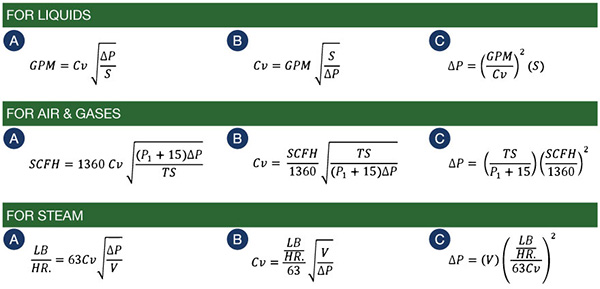### Flow Formulas

Valves with a "Full Port" have an internal seat diameter that is the same as the nominal pipe size, i.e. a 1 inch pipe size valve with a full port has a 1 inch diameter seat. Valves with a "Reduced Port" have an internal seat diameter that is smaller than the nominal pipe size.

The valve's flow coefficient, Cv, is a value that is determined by flow testing for each valve size. Full port valves will have a higher Cv than reduced port valves. The Cv rating for each valve is listed in the tables found in the valve catalog.

The definition of Cv is the # of gallons of water that will flow through the valve with a 1 PSI pressure differential when the valve is open.

The equations below can be used to determine:

• Flow Rate, given the Cv and ΔP
• Cv, given the Flow Rate and ΔP
• ΔP, given the Flow Rate and Cv

Symbols

• Cv = Valve's flow coefficient (dimensionless value)
• S = Specific Gravity (1.0 for air or water)
• T = Absolute Temperature in °R (°R = °F + 460)
• P1 = Inlet Pressure in PSIG
• ΔP = Pressure Differential in PSI across value in the open position
• V = Specific Volume in Cubic Feet per Pound